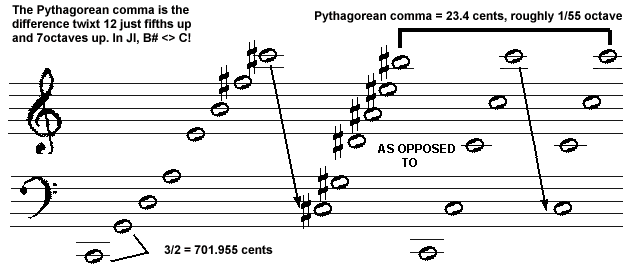# pythagorean-comma

[Brian McLaren]

Commas are small musical intervals between a note formed by one cycle of just intervals and the same note formed by another cycle of different just intervals. These commas prove important in constructing musical scales, and in notating musical chords and musical pitches.

The Pythagorean comma is the difference between 12 just perfect fifths up and 7 octaves up:. . . . . . . . .
[Joe Monzo]

McLaren's description can be shown in both monzo notation and in regular fractional math thus:

```    2,3-monzo

[-12 12, >        (3/2)12 = 312/212 = 531441/4096      531441/4096    12 "5ths"
- [  7  0, >      ÷ (2/1)7  = 128                     *      1/128      7 "8ves"
------------  =  ---------                        =  ---------------
[-19 12, >                                           531441/524288   Pythagorean comma
```
. . . . . . . . .

The ratio 531441/ 524288, in JustMusic prime-factor notation designated as 312, written in decimal form as exactly 1.0136432647705078125. Its logarithmic interval size is approximately 0.23 Semitones [= ~23.46001038 cents].

It is the difference between the pythagorean or 3-limit "tritone" or "augmented 4th" of approximately 6.12 Semitones [= 729/512 = 36 = ~611.7300052 cents] and the pythagorean or 3-limit diminished 5th of approximately 5.88 Semitones [= 1024/729 = 3-6 = ~588.2699948 cents], shown below in both vector notation and in regular fractional math:

```for C = n0 = 1/1 :

2,3-monzo

[ -9  6, >       (9/8)3          = 93/83           =  729/512       729/512      tritone: F:B
- [ 10 -6, >     ÷ (223-1)/(2-835)  = (4/3)/(243/256) = 1024/729    *  729/1024     "diminished 5th": B:F
-----------  =  ---------                                      =  -------------
[-19 12, >                                                       531441/524288   Pythagorean comma
```

The pythagorean comma was first described c. 300 BC by pseudo-Euclid in Divisions of the Canon.

Below are some other interval measurements for the Pythagorean comma:

```   ~0.115050808  (~3/26 = ~1/8) 9/8 ratio tone (i.e., ~1/8-tone)
~0.234600104  (~1/4) 12-edo Semitone
~1.407600623  (~1 2/5) 72-edo-moria
~5.865002596  (~5 6/7) savarts
~11.9646053 (~11 27/28) 612-edo schismas
~14.27150632  (~14 1/4) 730-edo Woolhouse-units
~19.55000865  (~19 5/9) millioctaves
~23.46001038  (~23 1/2) cents
~207.2300917  (~207 1/4) tÃ¼rk-sents
~588.5139105  (~588 1/2) jots
720.0000000 (720) temperament-units
~960.9220254  (~961 71/77) 12mus
~3843.688101  (~3843 2/3) 14mus
```
. . . . . . . . .

The standard epimoric approximation to the Pythagorean comma is the ratio 74:73 ( = ~23.5545681 cents), whose 2,3,37,73-monzo is 1, 0, 1, -1 > . The difference between them is:

```   -------- monzo --------
2    3  37  73

|  1    0   1  -1 >    =  epimoric approximation, 74:73 ratio
-  |-19   12   0   0 >    =  actual Pythagorean-comma,
----------------------
| 20  -12   1  -1 >    =  860535:860488 ratio = ~0.094557714  cent ( = ~1/10 or ~2/21 cent)
```

Tuning treatises before c.1970 often defined the Pythagorean-comma as the 74:73 ratio without comment on the fact of its being an approximation, particularly in the German literature of c.1850-1950. One example is Helmholtz in On the Sensations of Tone..., where Helmholtz mentions once that 74:73 is an approximation then proceeds to use it as the ratio of the pythagorean-comma.

. . . . . . . . .

The tonalsoft.com website is almost entirely the work of one person: me, Joe Monzo. Please reward me for my knowledge and effort by choosing your preferred level of financial support. Thank you.

 support level donor: \$5 USD friend: \$25 USD patron: \$50 USD savior: \$100 USD angel of tuning: \$500 USD microtonal god: \$1000 USD